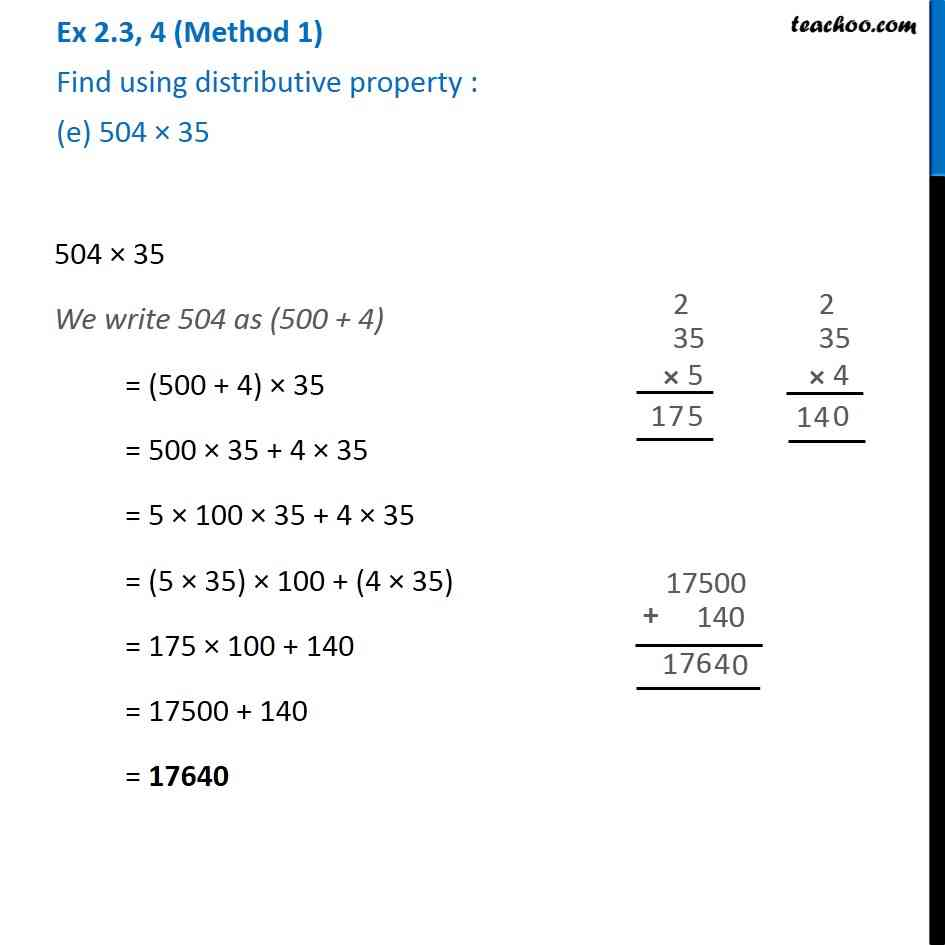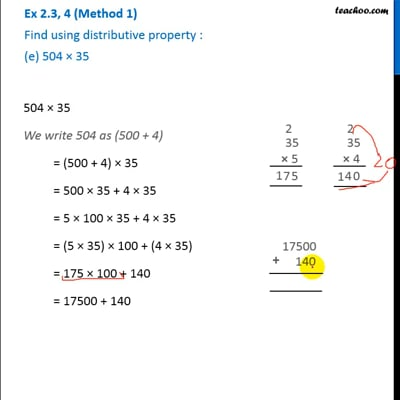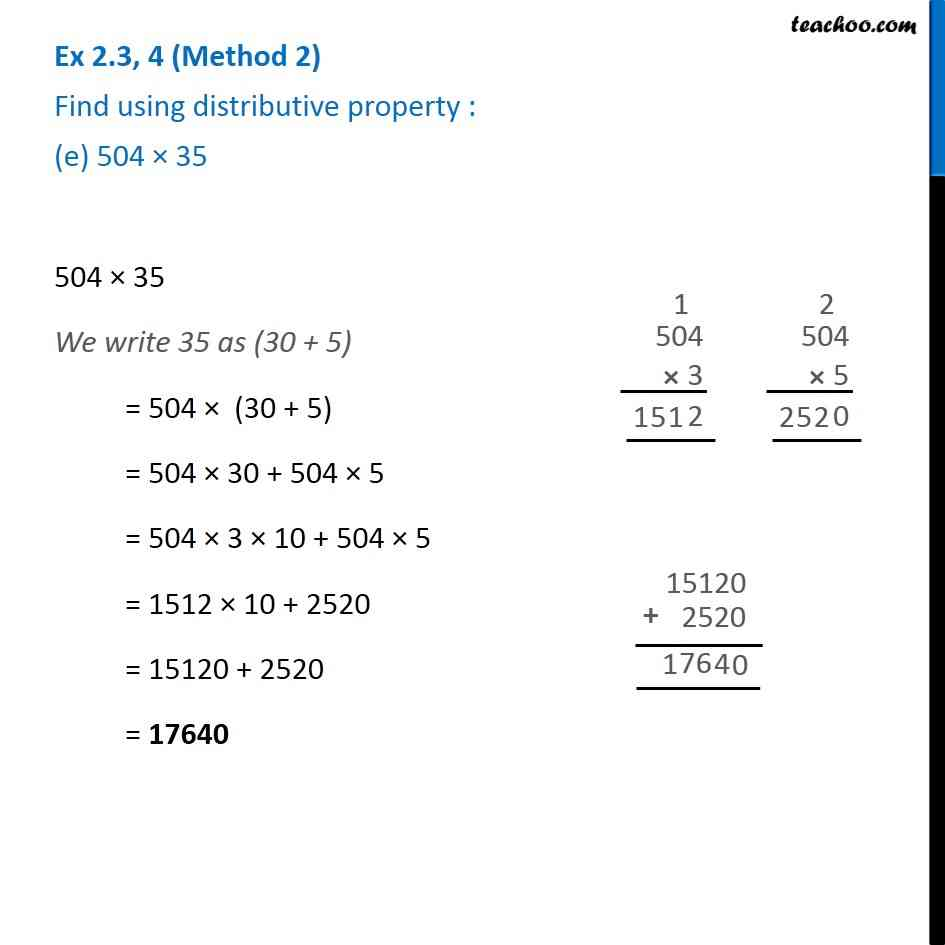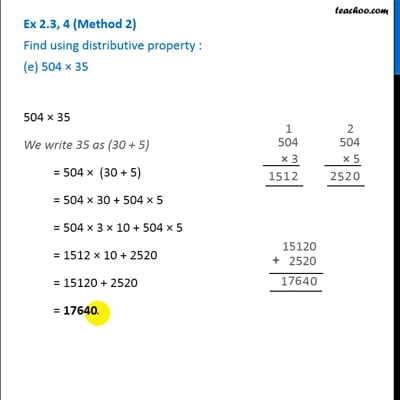Properties of Whole Numbers

Chapter 2 Class 6 Whole Numbers
Serial order wiseThis video is only available for Teachoo black usersThis video is only available for Teachoo black users

Learn in your speed, with individual attention - Teachoo Maths 1-on-1 Class

### Transcript

Question 4 (Method 1) Find using distributive property : (e) 504 × 35504 × 35 = (500 + 4) × 35 = 500 × 35 + 4 × 35 = 5 × 100 × 35 + 4 × 35 = (5 × 35) × 100 + 4 × 35 = 175 × 100 + 140 = 17500 + 140 = 17640 Question 4 (Method 2) Find using distributive property : (e) 504 × 35504 × 35 = 504 (30 + 5) = 504 × 30 + 504 × 5 = 504 × 3 × 10 + 504 × 5 = 1512 × 10 + 2520 = 15120 + 2520 = 17640